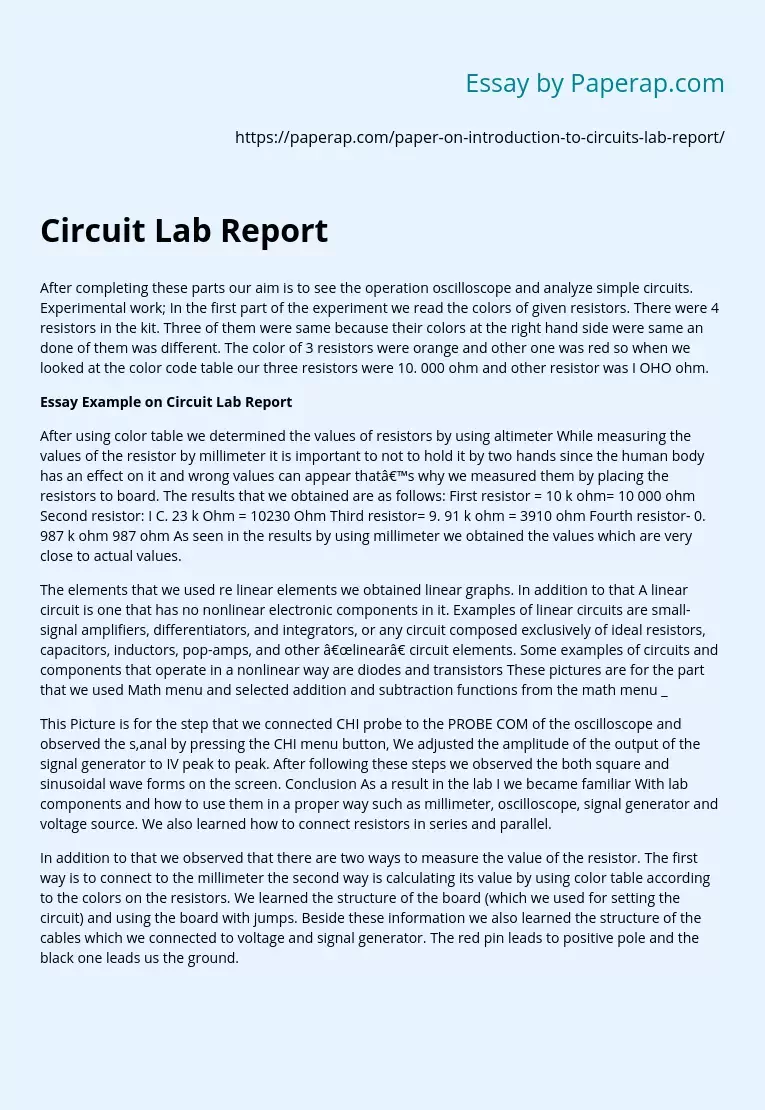# Circuit Lab Report

The following sample essay on after completing these parts our aim is to see the operation oscilloscope and analyze simple circuits. Experimental work; In the first part of the experiment we read the colors of given resistors. There were 4 resistors in the kit. Three of them were same because their colors at the right hand side were same an done of them was different. The color of 3 resistors were orange and other one was red so when we looked at the color code table our three resistors were 10.

000 ohm and other resistor was I OHO ohm.

## Essay Example on Circuit Lab Report

After using color table we determined the values of resistors by using altimeter While measuring the values of the resistor by millimeter it is important to not to hold it by two hands since the human body has an effect on it and wrong values can appear that’s why we measured them by placing the resistors to board.

The results that we obtained are as follows: First resistor = 10 k ohm= 10 000 ohm Second resistor: I C. 23 k Ohm = 10230 Ohm Third resistor= 9. 91 k ohm = 3910 ohm Fourth resistor- 0. 987 k ohm 987 ohm As seen in the results by using millimeter we obtained the values which are very close to actual values. The elements that we used re linear elements we obtained linear graphs. In addition to that A linear circuit is one that has no nonlinear electronic components in it. Examples of linear circuits are small-signal amplifiers, differentiators, and integrators, or any circuit composed exclusively of ideal resistors, capacitors, inductors, pop-amps, and other “linear” circuit elements.

Get quality help nowRhizManVerified

Proficient in: Electrical Engineering4.9 (247)

“ Rhizman is absolutely amazing at what he does . I highly recommend him if you need an assignment done ”+84 relevant experts are online

Some examples of circuits and components that operate in a nonlinear way are diodes and transistors These pictures are for the part that we used Math menu and selected addition and subtraction functions from the math menu. This Picture is for the step that we connected CHI probe to the PROBE COM of the oscilloscope and observed the s,anal by pressing the CHI menu button, We adjusted the amplitude of the output of the signal generator to IV peak to peak. After following these steps we observed the both square and sinusoidal wave forms on the screen. Conclusion As a result in the lab I we became familiar With lab components and how to use them in a proper way such as millimeter, oscilloscope, signal generator and voltage source. We also learned how to connect resistors in series and parallel. In addition to that we observed that there are two ways to measure the value of the resistor. The first way is to connect to the millimeter the second way is calculating its value by using color table according to the colors on the resistors. We learned the structure of the board (which we used for setting the circuit) and using the board with jumps. Beside these information we also learned the structure of the cables which we connected to voltage and signal generator. The red pin leads to positive pole and the black one leads us the ground.